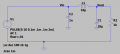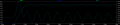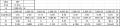# How to find Vpp of a inductor voltage from RCL resonance circuit.

#### justinjunghkim

Joined Dec 23, 2014
16
Hello,

I'm having a trouble to estimate Vpp of a RCL oscillation circuit. I found a peak to peak inductor voltage (Vpp_L) varies with the different combination of LC, which makes sense because the frequency (f) is different.
Then what is the relationship between Vpp_L and LC combination?

Here are things that I have done.

I noticed that the input battery voltage is just a constant voltage coef., meaning Vpp_L = Vbat x Coef_Vpp_L.
*Coef_Vpp_L: Coefficient of Vpp_L that I think it should be a combination with Cap and Inductor.

I used the following equations to solve this problem, but it wouldn't help. Because I have to know either a voltage or a current.
Vpp_L = 1/sqrt(C/L) x Ipp_L
Vpp_L = L * di/dt.
I = C*dV/dt.

Here are a circuit and its simulation result.I used 1kΩ for R2, so every time I change the cap, the output voltage wouldn't decrease as quickly.In transient, an inrush current is occurred, which is nothing to do with inductor current.

Vin = 10V
Vpp_L = Vout = 2V @ first wave.
Ipp_L = 2.9A
Resonance frequency, f = 11kHz.

Here is a measurement table. I changed the capacitance.I recorded dI from the simulation, f and VL are calculated.
What I don't understand here when C1 is 10uF the current is increased..